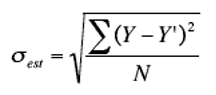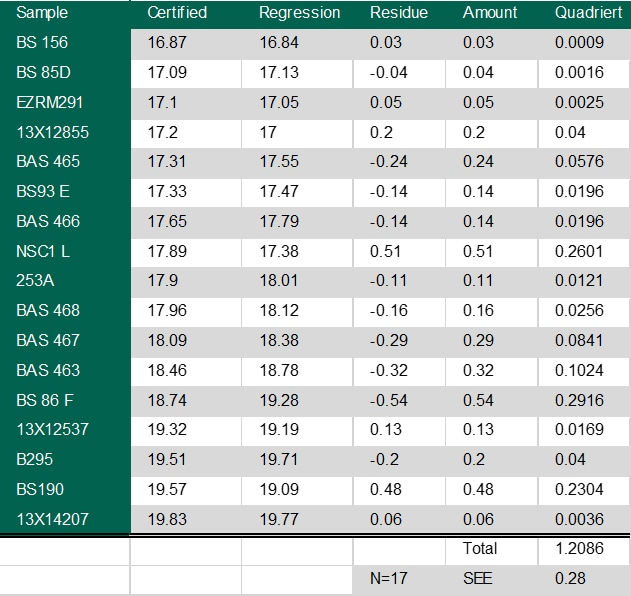# Calculate Measurement Uncertainty without a Reference Sample

GUM, the internationally approved technique for calculating measurement uncertainty, is reliant on the availability of a certified reference sample. Likewise, to find any repeatable offset (systematic deviation of the mean) of an analyzer, reference samples are also utilized.

What can you do when you do not have a reference sample, or the one you do possess is not within the region of interest for your current measurement? You can circumvent this by applying different statistical techniques. Before outlining the techniques, measurement uncertainty is described in more detail below.

## Components of Measurement Uncertainty: Trueness and Precision

An accurate reading must fulfill two criteria:

### Precision

If the same measurement is taken multiple times, precision is a measure of how similar the results are. Poor precision exhibits a big fluctuation every time, but good precision yields results which are close together. Precision is a result of random fluctuations, so the results are of a normal distribution.

### Accuracy of the Mean (Trueness)

This is how much the mean of the measurements deviates from the expected result. Measurement errors as a result of this are seen as a systematic deviation of the mean for every measurement made in the instrument.

To get an overall confidence interval for accuracy, both of these must be calculated and then the results combined.

## Calculating Accuracy of the Mean Without a Reference

If a reference sample of known composition was possessed, the certified value could be compared to the averaged measured values and the resulting offset applied to all of the results. If that option is not available, another technique must be used – the Standard Error of the Estimate or SEE.

The equation for the standard error of the estimate is shown below:Where:

σest is the standard error of the estimate
Y is the actual result measured by the instrument
Y’ is the result after regression analysis
N is the number of values

If some real numbers are used it is easier to see how this works. Below is a table of results from a spectrometer.The measured values can be seen in the first column of figures, the second is the result after the software has applied the regression analysis. Employing these numbers in the above formula generates a standard error of the estimate of 0.28%.

## Calculating Precision Without a Reference

When the GUM technique for measurement uncertainty cannot be employed due to random fluctuations, the Student t-distribution function is used. This distribution function was developed when the amount of samples is extremely low.

The confidence interval given by the Student t-distribution function is given by:

uc =  X̅ +/- T

Where:

X̅ : average of the measured values
TX : value of the t-distribution function. This is calculated from the following formula:

Tx = (t (f,P) x s / N1/2

Where:

t : value taken from published tables which depends on f (number of samples measured - 1) and P (the desired confidence level)
s : standard deviation of the measurement series
N : number of measurements taken

If we assume we have measured an unknown sample 10 times and have the following results for chromium composition:

Average composition from 10 readings: 18.82%
Standard deviation 0.15%

We can work within a confidence level of 95%, which means that the values we need to put into the above formulae are:

n : 10
P : 95%
s : 0.15%
t : 2.262 (for P of 95% and sample size 10)

Working using these numbers supplies us with a confidence level.

Uc = +/- 0·11%

## Combining Precision and Trueness

Now we have everything required to calculate the overall measurement uncertainty. All that is left is to add the value of +/- 0.11% for precision to the value of +/- 0.28 for accuracy of the mean to get the final result:

C = 18.82% +/- 0.39% @ 95% probability.This information has been sourced, reviewed and adapted from materials provided by Hitachi High-Tech Analytical Science.

## Citations

• APA

Hitachi High-Tech Analytical Science. (2020, January 15). Calculate Measurement Uncertainty without a Reference Sample. AZoM. Retrieved on July 06, 2020 from https://www.azom.com/article.aspx?ArticleID=18469.

• MLA

Hitachi High-Tech Analytical Science. "Calculate Measurement Uncertainty without a Reference Sample". AZoM. 06 July 2020. <https://www.azom.com/article.aspx?ArticleID=18469>.

• Chicago

Hitachi High-Tech Analytical Science. "Calculate Measurement Uncertainty without a Reference Sample". AZoM. https://www.azom.com/article.aspx?ArticleID=18469. (accessed July 06, 2020).

• Harvard

Hitachi High-Tech Analytical Science. 2020. Calculate Measurement Uncertainty without a Reference Sample. AZoM, viewed 06 July 2020, https://www.azom.com/article.aspx?ArticleID=18469.